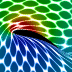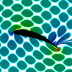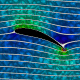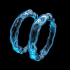## Archive for the 'potential flow' Category

17
Nov
07

### Periodic Steady Vortices in a Stagnation Point FlowPeriodic Steady Vortices in a Stagnation Point Flow. I got the idea for this potential flow animation from this paper. The entrained fluid was integrated using the 4th order Runge-Kutta method.
Advertisements
11
Nov
07

### Schwarz-Christoffel FlowHere’s a potential flow of a vortex near some stair steps. This was accomplished by mapping a flat wall to stair steps using a Schwarz-Christoffel transformation:

```(* runtime: 0.7 second *) Clear[z]; gamma = 1; z0 = 1 + I; z1 = I;z2 = 0; z3 = 1; zeta1 = -1; zeta2 = 0; zeta3 = 1; theta1 = 3Pi/2; theta2 = Pi/2; theta3 = 3Pi/2; z = z[zeta] /. DSolve[{z'[zeta] == K(zeta - zeta1)^(theta1/Pi - 1)(zeta - zeta2)^(theta2/Pi - 1)(zeta -zeta3)^(theta3/Pi - 1), z[zeta1] == z1}, z[zeta], zeta][]; z = z /. K -> (K /. Solve[(z /. zeta -> zeta2) == z2, K][]); zeta0 = zeta /. FindRoot[z == z0, {zeta, zeta2 + I}]; Z[w_] = z /. zeta -> (zeta /. Solve[w == (I gamma/(2Pi))(Log[zeta - zeta0] - Log[zeta - Conjugate[zeta0]]), zeta][]); ToPoint[z_] := {Re[z], Im[z]}; grid = Table[ToPoint[Z[phi + I psi]], {phi, -1, 0, 0.05}, {psi, -1*^-6, -1, -0.02}]; Show[Graphics[{Map[Line, grid], Map[Line, Transpose[grid]]}, AspectRatio -> Automatic, PlotRange -> {{-1, 2}, {-1, 2}}]]```

08
Nov
07

### Joukowski AirfoilThese animations were created using a conformal mapping technique called the Joukowski Transformation. A Joukowski airfoil can be thought of as a modified Rankine oval. It assumes inviscid incompressible potential flow (irrotational). Potential flow can account for lift on the airfoil but it cannot account for drag because it does not account for the viscous boundary layer (D’Alembert’s paradox). In these animations, red represents regions of low pressure. The left animation shows what the surrounding fluid looks like when the Kutta condition is applied. Notice that the fluid separates smoothly at the trailing edge of the airfoil and a low pressure region is produced on the upper surface of the wing, resulting in lift. The lift is proportional to the circulation around the airfoil. The right animation shows what the surrounding fluid looks like when there is no circulation around the airfoil (stall). Notice the sharp singularity at the trailing edge of the airfoil.Here is an animation that shows how the streamlines change when you increase the circulation around the airfoil. (Please note: The background fluid motion in this animation is just for effect and is not accurate!) Here is some Mathematica code to plot the streamlines and pressure using Bernoulli’s equation:
```(* runtime: 13 seconds *) U = rho = 1; chord = 4; thk = 0.5; alpha = Pi/9; y0 = 0.2; x0 = -thk/5.2; L = chord/4; a = Sqrt[y0^2 + L^2]; gamma = 4Pi a U Sin[alpha + ArcCos[L/a]]; w[z_, sign_] := Module[{zeta = (z + sign Sqrt[z^2 - 4 L^2])/2}, zeta = (zeta - x0 - I y0)Exp[-I alpha]/Sqrt[(1 - x0)^2 + y0^2]; U(zeta + a^2/zeta) + I gamma Log[zeta]/(2Pi)]; sign[z_] := Sign[Re[z]]If[Abs[Re[z]] < chord/2 && 0 < Im[z] < 2y0(1 - (2Re[z]/chord)^2), -1, 1];w[z_] := w[z, sign[z]]; V[z_] = D[w[z, sign], z] /. sign -> sign[z]; << Graphics`Master`; DisplayTogether[DensityPlot[-0.5rho Abs[V[(x + I y)Exp[I alpha]]]^2, {x, -3, 3}, {y, -3, 3}, PlotPoints -> 275, Mesh -> False, Frame -> False, ColorFunction -> (If[# == 1, Hue[0, 0, 0], Hue[(5# - 1)/6]] &)],ContourPlot[Im[w[(x + I y)Exp[I alpha]]], {x, -3, 3}, {y, -3, 3}, Contours -> Table[x^3 + 0.0208, {x, -2, 2, 0.1}], PlotPoints -> 100, ContourShading -> False], AspectRatio -> Automatic];```

24
Oct
07

### Magnus EffectA Curveball is a spinning ball that accelerates sideways due to the Magnus Effect. Here is some Mathematica code to plot the streamlines around a lifting cylinder, assuming inviscid incompressible potential flow (irrotational) :
```(* runtime: 0.3 second *) U = 1; gamma = 4; a = 1; w[z_] := U(z + a^2/z) + I gamma Log[z]/(2Pi); ContourPlot[Im[w[x + I y]], {x, -2, 2}, {y, -2, 2}, Contours -> Table[psi, {psi, -3, 3, 0.25}], PlotPoints -> 100, ContourShading -> False, AspectRatio -> Automatic]```

Here is some Mathematica code to plot the entrained fluid using the 4th order Runge-Kutta method:
```(* runtime: 17 seconds, increase n for better resolution *) Clear[v]; n = 40; U = 1; gamma = 4; a = 1; tmax = 5.0; dt = tmax/100; v[z_] := If[Abs[z] < 1, 0, Conjugate[U (1 - (a/z)^2) + I gamma/(2Pi z)]]; image = Table[z = x + I y; Do[k1 = dt v[z]; k2 = dt v[z + k1/2]; k3 = dt v[z + k2/2]; k4 =dt v[z + k3]; z -= (k1 + 2 k2 + 2 k3 + k4)/6, {t, tmax, 0, -dt}]; z, {y, -2, 2, 4.0/n}, {x, -2, 2, 4.0/n}]; ListDensityPlot[Map[Abs[Mod[#, 1]] &, image, {2}], Mesh -> False, Frame -> False, AspectRatio -> 1]```

Link: My Educational Project – some basic potential flows using Matlab and Mathematica

31
Dec
05

### Leap-Frogging Bubble RingsHere is a simple technique for modeling vortices assuming inviscid incompressible potential flow (irrotational). This technique was inspired from Kerry Mitchell’s paper based on Ultra Fractal. You can also see this here on Dynamical Systems’ Picture Gallery.

Here is some Mathematica code to plot the entrained fluid:
``` (* runtime: 25 seconds, increase n for better resolution *) tmax = 0.85; rcore = 0.1; Klist = {1, -1, 1, -1}; zlist0 = {-1 - 0.5I, -1 + 0.5I, -0.5 - 0.5I, -0.5 + 0.5I}; m = Length[zlist0]; v[K_, z_, z0_] := Module[{r2 = Abs[z - z0]^2}, I K(z0 - z)/r2(1 - Exp[-r2/rcore^2])]; zlist = NDSolve[Flatten[Table[{Subscript[z,i]'[t] == Sum[If[i == j, 0, v[Klist[[j]], Subscript[z, i][t], Subscript[z, j][t]]], {j, 1, m}], Subscript[z, i] ==zlist0[[i]]}, {i, 1, m}]], Table[Subscript[z, i][t], {i, 1, m}], {t, 0, tmax}][[1, All, 2]]; n = 23; image = Table[NDSolve[{z'[t] == Sum[v[Klist[[i]], z[t], zlist[[i]]], {i, 1, m}], z[tmax] == x + I y}, z[t], {t, 0, tmax}, MaxSteps -> 5000][[1, 1, 2]] /. t -> 0, {y, -1.125, 1.125, 2.25/n}, {x, -0.35, 1.9, 2.25/n}]; ListDensityPlot[Map[Sign[Im[#]]Arg[#] &, image, {2}], Mesh -> False, Frame -> False, AspectRatio -> Automatic]```

16
Dec
05

### NACA AirfoilHere is a 9415 NACA airfoil. It’s almost shaped the same as the Joukowski airfoil (but it’s a little different):
```(* runtime: 0.01 second *) n = 40; c = 0.09; p = 0.4; t = 0.15; Clear[x]; camber := c If[x < p, (2p x - x^2)/p^2, ((1 - 2p) + 2p x - x^2)/(1 - p)^2]; theta = ArcTan[D[camber, x]]; p = Table[x = 0.5(1 - Cos[Pi s]); x1 = 1.00893x; thk = 5t(0.2969Sqrt[x1] - 0.126x1 - 0.3516x1^2 + 0.2843x1^3 - 0.1015x1^4); {x, camber} + Sign[s]thk {-Sin[theta],Cos[theta]}, {s, -1, 1, 2/(n - 1)}]; ListPlot[p, PlotJoined -> True, AspectRatio -> Automatic];```

We can approximate the pressure profile using the vortex panel method assuming inviscid incompressible potential flowirrotational). The following Mathematica code was adapted from my Fortran program for my 4/25/99 research project on optimal airfoil design:``` (* runtime: 0.3 second *) alpha = Pi/9; pc = Table[(p[[i]] + p[[i + 1]])/2, {i, 1, n - 1}]; s = Table[v = p[[i + 1]] - p[[i]];Sqrt[v.v], {i, 1, n - 1}]; theta = Table[v = p[[i + 1]] - p[[i]]; ArcTan[v[], v[]], {i, 1, n - 1}]; sin = Sin[theta]; cos = Cos[theta]; Cn1 = Cn2 = Ct1 = Ct2 =Table[0, {n - 1}, {n - 1}]; Do[If[i == j, Cn1[[i, j]] = -1; Cn2[[i, j]] = 1; Ct1[[i, j]] = Ct2[[i, j]] = Pi/2,v = pc[[i]] - p[[j]]; a = -v.{cos[[j]], sin[[j]]}; b = v.v;t = theta[[i]] - theta[[j]];c = Sin[t]; d = Cos[t]; e = v.{sin[[j]], -cos[[j]]}; f =Log[1 + s[[j]](s[[j]] + 2a)/b]; g = ArcTan[b + a s[[j]], e s[[j]]];t = theta[[i]] - 2theta[[j]]; p1 = v.{Sin[t], Cos[t]}; q = v.{Cos[t], -Sin[t]}; Cn2[[i, j]] = d + (0.5q f - (a c + d e)g)/s[[j]]; Cn1[[i, j]] = 0.5d f + c g - Cn2[[i, j]];Ct2[[i, j]] = c + 0.5p1 f/s[[j]] + (a d - c e)g/s[[j]]; Ct1[[i, j]] = 0.5c f - d g - Ct2[[i, j]]], {i, 1, n - 1}, {j, 1, n - 1}]; gamma = LinearSolve[Table[If[i == n, If[j == 1 || j == n, 1, 0], If[j == n, 0, Cn1[[i, j]]] + If[j == 1, 0, Cn2[[i, j - 1]]]], {i, 1, n}, {j, 1, n}], Table[If[i ==n, 0, Sin[theta[[i]] - alpha]], {i, 1, n}]]; ListPlot[Table[q = Cos[theta[[i]] - alpha] + Sum[(If[j == n, 0, Ct1[[i, j]]] + If[j == 1, 0, Ct2[[i, j - 1]]])gamma[[j]], {j, 1, n}]; {pc[[i, 1]], 1 - q^2}, {i, 1, n - 1}], PlotJoined -> True];```

Here is some code for a pretty pressure plot:
```(* runtime: 33 seconds *) w[z_] := z Exp[-I alpha] + I Sum[s[[j]]gamma[[j]]Log[z - pc[[j, 1]] - I pc[[j, 2]]], {j, 1, n - 1}]; V[z_] = D[w[z], z]; DensityPlot[-Abs[V[(x + I y)Exp[I alpha]]]^2, {x, -0.25, 1.25}, {y, -0.75, 0.75}, PlotPoints -> 275, Mesh -> False, Frame -> False, ColorFunction -> (Hue[(5# - 1)/6] &), AspectRatio -> Automatic];```

## Welcome !

You will find here some of my favorite hobbies and interests, especially science and art.

I hope you enjoy it!

Subscribe to theRSS feed to stay informed when I publish something new here.

I would love to hear from you! Please feel free to send me an email : bugman123-at-gmail-dot-com

## Recent CommentsBerna Blalack on Magnetic Pendulum Strange…Daan on Magnetic Pendulum Strange…Sebastian Schepis on Diffusion Limited Aggregation…mohammad_andito on CFM56-5 Turbofan Jet Engi…SasQ on Magnetic Field of a Solen… OUPblog » Blog… on Diamond Light Dispersion Complex Roots on Polynomial Roots Joukowski airfoils |… on Joukowski AirfoilKarim Alame on Flapping WingREJISH J on Joukowski Airfoil SOLINOID | Materials… on Magnetic Field of a Solen…Emanuele on 4D “Squarry” Julia…Emanuele on Hydrogen Electron Orbital Prob…Tim on Mandelbrot Set Pickover S…khankasi1 on 4D Quaternion Mandelbrot …

• 561,072 hits
Advertisements